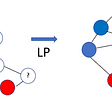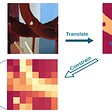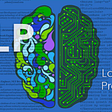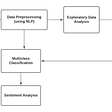# Natural Language Processing: Text Data Vectorization

Features in machine learning is basically numerical attributes from which anyone can perform some mathematical operation such as matrix factorisation, dot product etc. But there are various scenario when dataset does not contain numerical attribute for example- sentimental analysis of Twitter/Facebook user , Amazon customer review , IMDB/Netflix movie recommendation . In all the above cases dataset contain numerical value, string value, character value, categorical value, connection (one user connected to another user). Conversion of these types of feature into numerical feature is called featurization

In this chapter I will discuss how to convert string features into numerical features. Let us consider following review

Figure 1 and Figure 2 represent different user review for a product. We can use these types of reviews in a dataset and predict the sentiment of user. But these features are in the form of string so first we need to convert these string features into numerical features. To convert string data into numerical data one can use following methods

· Bag of words

· TFIDF

· Word2Vec

Text Preprocessing

Raw data contain numerical value, punctuation,special character etc as shown in Figure 1 and Figure 2 . These value can hamper the performance of model so before applying any text featurization first we need to convert raw data into meaningful data which is also called as text preprocessing . This can be done by following ways:

1 -Remove Noisy Data

In regular sentences Noisy data can be defined as text file header,footer, HTML,XML,markup data.As these type of data are not meaningful and does not provide any information so it is mandatory to remove these type of noisy data. In python HTML,XML can be removed by BeautifulSoup library while markup,header can be removed by using regular expression

2- Tokenization

In tokenization we convert group of sentence into token . It is also called text segmentation or lexical analysis. It is basically splitting data into small chunk of words. For example- We have sentence — “Ross 128 is earth like planet.Can we survive in that planet?”. After tokenization this sentence will become -[‘Ross’, ‘128’, ‘is’, ‘earth’, ‘like’, ‘planet’, ‘.’, ‘Can’, ‘we’, ‘survive’, ‘in’, ‘that’, ‘planet’, ‘?’]. Tokenization in python can be done by python’s NLTK library’s word_tokenize() function

3- Normalization

Before going to normalization first closely observe output of tokenization. Will tokenization output can be considered as final output? Can we extract more meanigful information from tokenize data ?

In tokenaization we came across various words such as punctuation,stop words(is,in,that,can etc),upper case words and lower case words.After tokenization we are not focused on text level but on word level. So by doing stemming,lemmatization we can convert tokenize word to more meaningful words . For example — [‘‘ross’, ‘128’, ‘earth’, ‘like’, ‘planet’ , ‘survive’, ‘planet’]. As we can see that all the punctuation and stop word is removed which makes data more meaningful

Bag of Words

It is basic model used in natural language processing. Why it is called bag of words because any order of the words in the document is discarded it only tells us weather word is present in the document or not , Let us understand Bag of word with following example

“There used to be Stone Age”

“There used to be Bronze Age”

“There used to be Iron Age”

“There was Age of Revolution”

“Now it is Digital Age”

Here each sentence is separate document if we make list of the word such that one word should be occur only once than our list looks like as follow:

“There”,”was”,”to”,”be”,”used”,”Stone”,”Bronze,”Iron”,”Revolution”,”Digital”,”Age”,”of”,”Now”,”it”,”is”

So how a word can be converted to vector can be understood by simple word count example where we count occurrence of word in a document w.r.t list. For example- vector conversion of sentence “There used to be Stone Age” can be represented as :

“There” = 1

”was”= 0

”to”= 1

”be” =1

”used” = 1

”Stone”= 1

”Bronze” =0

“Iron” =0

”Revolution”= 0

”Digital”= 0

”Age”=1

”of”=0

”Now”=0

”it”=0

”is”=0

So here we basically convert word into vector . By following same approach other vector value are as follow:

“There used to be bronze age” = [1,0,1,1,1,0,1,0,0,0,1,0,0,0,0]

“There used to be iron age” = [1,0,1,1,1,0,0,1,0,0,1,0,0,0,0]

“There was age of revolution” = [1,1,0,0,0,0,0,0,1,0,1,1,0,0,0]

“Now its digital Age” = [0,0,0,0,0,0,0,0,0,1,1,0,1,1,1]

The approach which is discussed above is unigram because we are considering only one word at a time . Similarly we have bigram(using two words at a time- for example — There used, Used to, to be, be Stone, Stone age), trigram(using three words at a time- for example- there used to, used to be ,to be Stone,be Stone Age), ngram(using n words at a time)

Hence the process of converting text into vector is called vectorization.

By using CountVectorizer function we can convert text document to matrix of word count. Matrix which is produced here is sparse matrix. By using CountVectorizer on above document we get 5*15 sparse matrix of type numpy.int64.

After applying the CountVectorizer we can map each word to feature indices as shown in figure 3

This can be transformed into sparse matrix by using as shown in figure 4

Note — Countvectorizer produces sparse matrix which sometime not suited for some machine learning model hence first convert this sparse matrix to dense matrix then apply machine learning model

TF-IDF

TF-IDF stands for Term Frequency-Inverse Document Frequency which basically tells importance of the word in the corpus or dataset. TF-IDF contain two concept Term Frequency(TF) and Inverse Document Frequency(IDF)

Term Frequency

Term Frequency is defined as how frequently the word appear in the document or corpus. As each sentence is not the same length so it may be possible a word appears in long sentence occur more time as compared to word appear in sorter sentence. Term frequency can be defined as:

Let’s understand with this example

Suppose we have sentence “The TFIDF Vectorization Process is Beautiful Concept” and we have to find the find frequency count of these words in five different documents

As shown in Table 1 frequency of ‘The’ is maximum in every Document. Suppose frequency of ‘The’ in Document6 is 2 million while frequency of ‘The’ in Document7 in 3 million. Frequency of ‘The’ is very large in Document6 and Document7 so we can add log term to reduce the value of frequency count (log(2 million) =21). Adding log not only dampen the performance of idf but also reduce the frequency count of TF. Hence formula of TF can be defined as:

When tf = 1 log term will become zero and value will become 1 . Adding 1 is just to differentiate between tf=0 and tf =1

Hence Table 1 can be modified to :

Inverse Document Frequency

Inverse Document frequency is another concept which is used for finding out importance of the word. It is based on the fact that less frequent words are more informative and important. IDF is represented by formula:

Let us consider the above example again

In Table 3 most frequent word is ‘The’ and ‘is ’ but it is least important according to IDF and the word which appear very less such as ‘TFIDF’, ‘Concept’ are important words. Hence, we can say that IDF of rare term is high and IDF of frequent term is low

TF-IDF

TF-IDF is basically a multiplication between Table 2 (TF table) and Table 3(IDF table) . It basically reduces values of common word that are used in different document. As we can see that in Table 4 most important word after multiplication of TF and IDF is ‘TFIDF’ while most frequent word such as ‘The’ and ‘is’ are not that important

Cosine Similarity

Before understanding word2vec lets first understand what is cosine similarity because word2vec uses cosine similarity for finding out most similar word (which will discussed in next section). Cosine similarity is not only telling the similarity between two vectors but it also test for orthogonality of vector. Cosine similarity is represented by formula:

Where theta is angle between two vector if angle are close to zero than we can say that vectors are very similar to each other and if theta is 90 than we can say vectors are orthogonal to each other (orthogonal vector not related to each other ) and if theta is 180 we can say that both the vector are opposite to each other as shown in figure 5

Cosine similarity not only finds out similarity between vector but it also ignore frequency count of word. Suppose word ‘The’ appear in Document1 200 times and in Document2 500 times but angle between both the words will be very close

It is deep learning technique with two-layer neural network.Google Word2vec take input from large data (in this scenario we are using google data) and convert into vector space. Google word2vec is basically pretrained on google dataset. To download the dataset you can follow this link — Google Dataset. So before diving into Google word2vec lets first understand what is word2vec.

Word2vec basically place the word in the feature space is such a way that their location is determined by their meaning i.e. words having similar meaning are clustered together and the distance between two words also have same meaning. Consider an example given below:

In the figure 6 when we are looking into Male-Female graph we are observing that distance between man and woman is same as distance between king (male) and queen (woman) Not only different gender but if we look into same gender we observe that distance between queen and woman and distance between king and man are same(king and man, queen and woman represent same gender comparison hence they must be equal distance)

Word2vec not only map meaningful words but it also map grammar also which is shown in Verb tense graph. In this graph Verb (walking and swimming) and Tense are equal distance to each other.

Similarly it can map Country with its capital as shown in Country-Capital graph.

For Google Word2vec we are using google dataset to train the model because not only it cover most of the words but it is also used by many machine learning guru’s/expert.

But before using Google word2vec we must install and import genism. Gensim is a robust open-source vector space modeling and topic modeling toolkit implemented in Python. To install the genism please go through this link — Gensim Installation

Model Implementation

First step in model implementation is to train the model and to training the model just follow simple command

Where keyedVectors is a package present inside genism and ‘GoogleNews-vectors-negative300.bin’ is a google dataset, which is used here for training

Now Suppose we have to find the most similar item present in dataset w.r.t. our chosen word. For example, we have to find all the similar word related to ‘Robots’ in google dataset:

Similarly word similar to ‘Man’

Here most_similar function is calculating cosine similarity between every words

References

--

--

--

## More from Paritosh Pantola

Love podcasts or audiobooks? Learn on the go with our new app.

## A Deep Dive into Stacking Ensemble Machine Learning — Part III## I do not understand t-SNE — Part 1## Splitting your data to fit any machine learning model## Smart Labeling Techniques:## Unearthing Value in Planet Data: Bridging the Gap Between Geospatial Data and Machine Learning## Guided Super-Resolution as Pixel-to-Pixel Transformation## Guide to Restricted Boltzmann Machines (RBM)— Part 2## NLP : Natural Language Processing